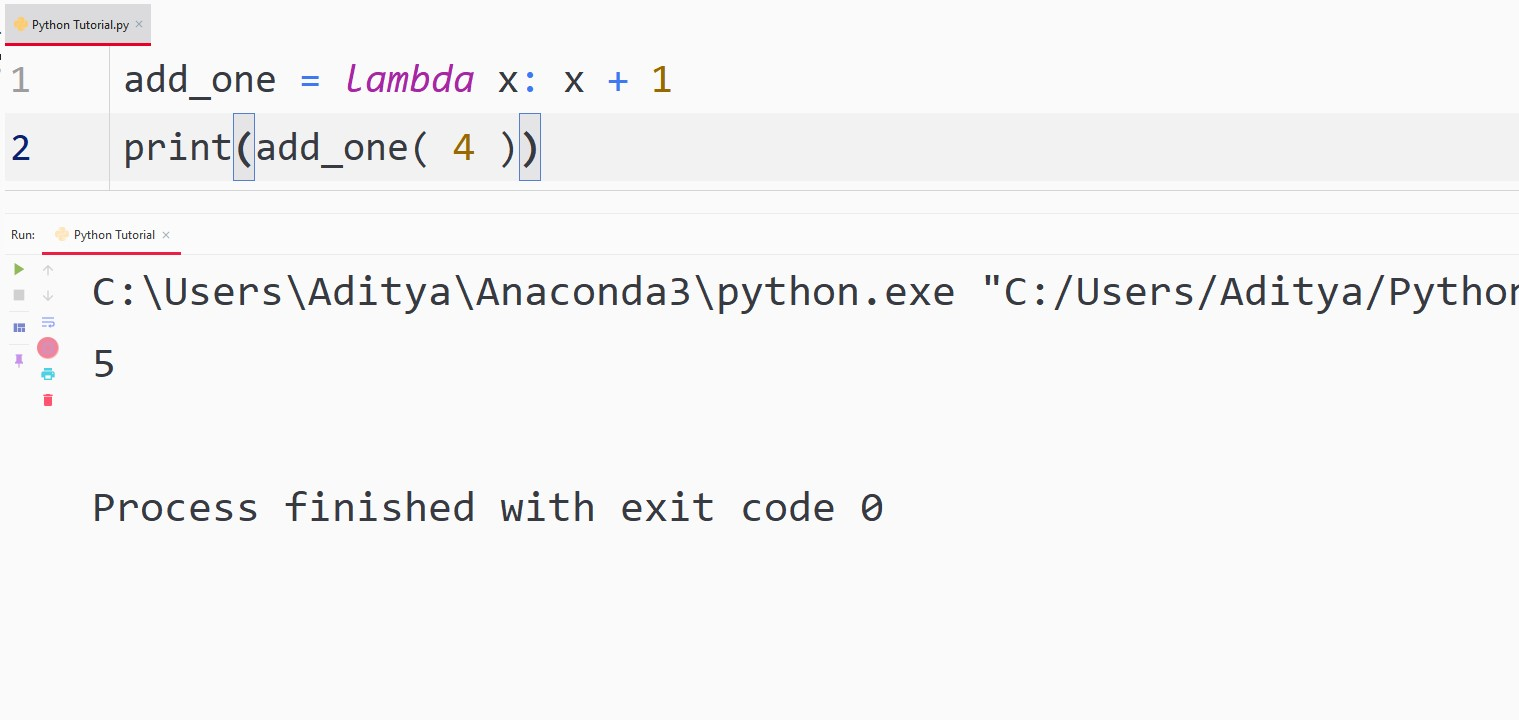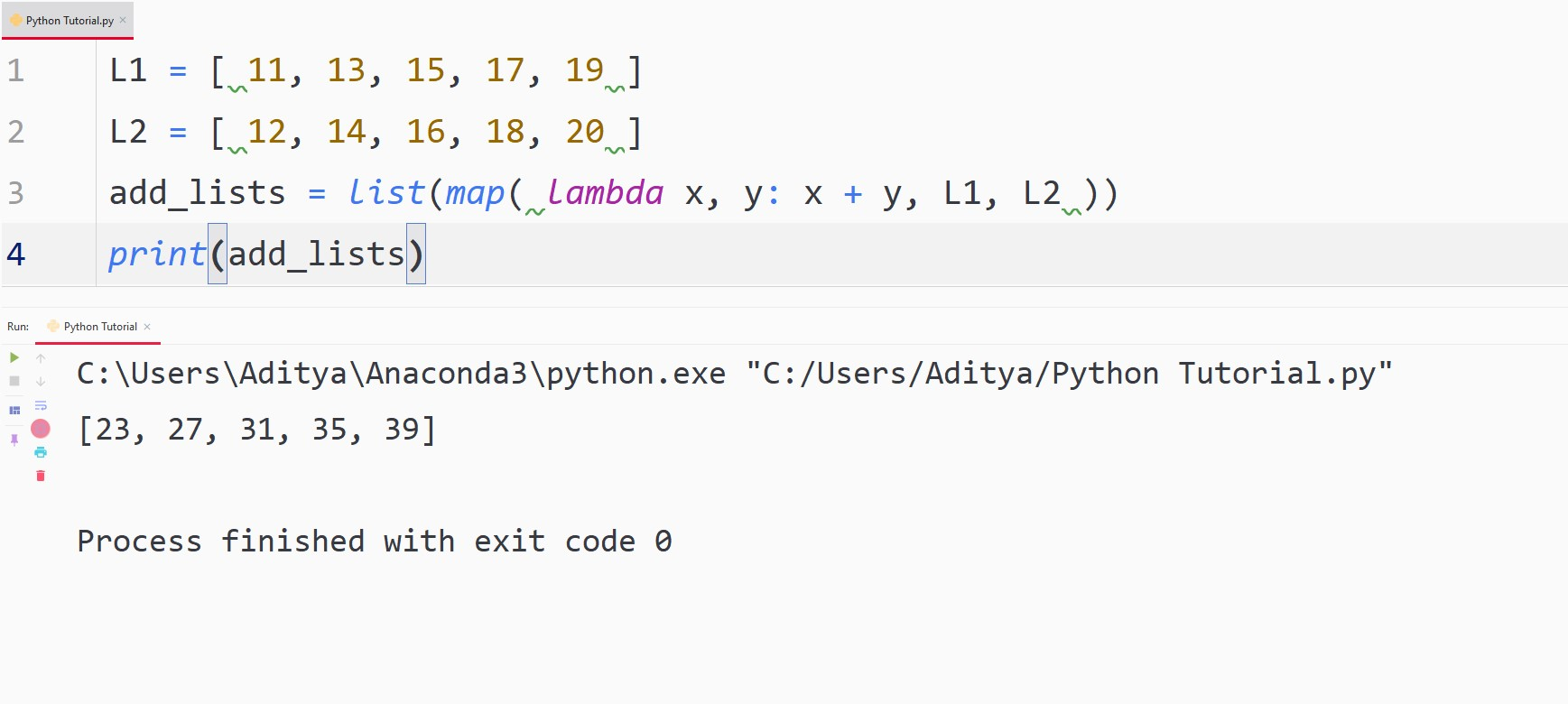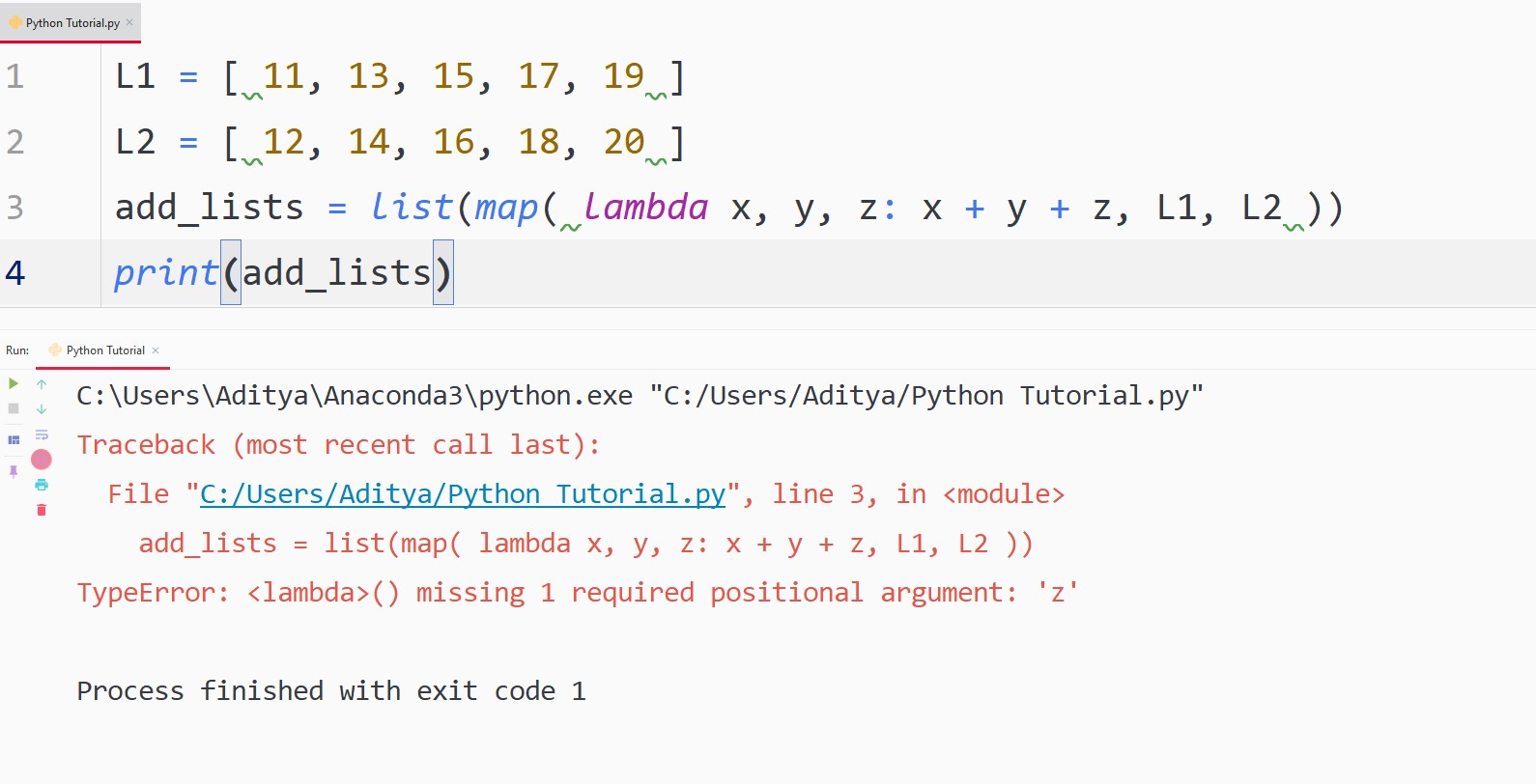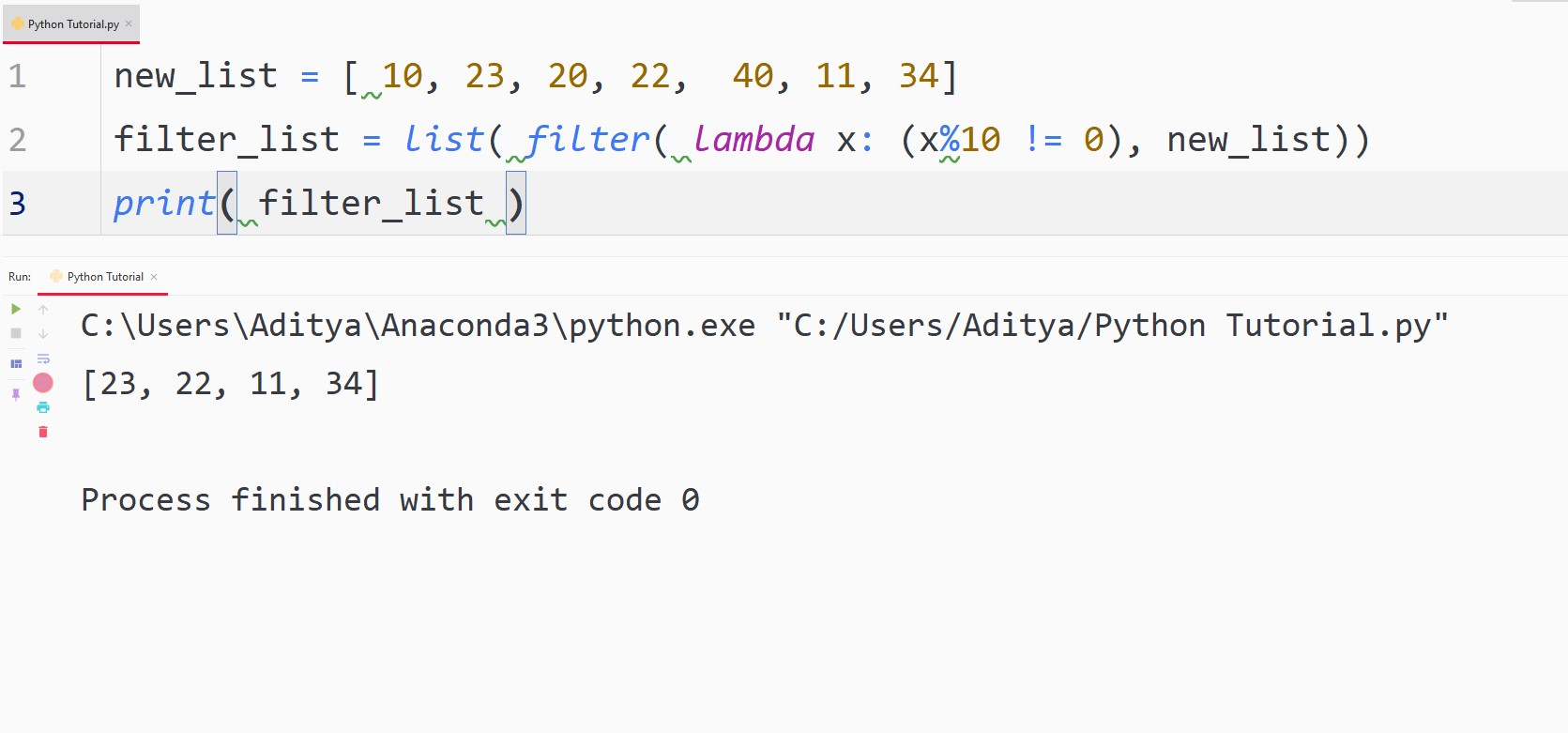# Python Lambda

## Python Lambda

Python Lambda is used to create anonymous functions. An anonymous function is a function in Python without a name.

To understand Python Lambda better, you need to know what the terms argument and expression mean in a function:

• An argument in a function is known as the values that are passed into the function or in other words arguments are the values that the function is allowed to work on.

An expression in a function is known as the task a function has to perform.

Now that we know the meaning of arguments and expressions, let us see what is Lambda:

Lambda is a keyword that is used to make anonymous functions. Unlike normal functions that are defined by using the keyword def, lambda functions, or anonymous functions are defined using the keyword lambda.

Syntax
lambda arguments: expression

The lambda function can have multiple arguments but can only have one expression

## ExampleAlso, the number of arguments can be more than one, and it can be seen in the example below –

## Example## Lambda with no Arguments

You can surely create a lambda function in Python with no arguments, however, it will serve no purpose whatsoever, because there will be no arguments to work on.

Let’s see how can you create one –

## ExampleYou can argue that it is serving the purpose of returning the square of two, but while coding you should look for the most efficient way to get the job done. The same thing can be done by declaring a variable and then printing it.

## Map() for Lambda

The map() function in Python takes another function and any number of sequences as arguments, for example, list, tuple, etc. It iterates through each element in the sequence and performs the operation of the function on them and then returns a list.

Normally, the function is a lambda and the code for map() can be written like this:

## ExampleYou can also pass multiple sequences as arguments in the map() functions until the number of sequences passed match the number of arguments passed in the lambda function.

## ExampleHowever, when the number of arguments in lambda and the number of sequences passed in the map() doesn’t match, the Python interpreter will give you an error.

## Example## Filter() for Lambda

The filter() function in Python for lambdas takes another function and a sequence kind of similar to the map() function above.

The function then iterates through all of the elements in the list and then returns a list that contains all of the elements for which the function evaluation was True.

Here, take an example of the filter() function which is filtering out all of the numbers that are not divisible by 10.

## ExampleThe real value of lambda can be see above using map() and filter() functions, they are very useful when you solve competitive programming questions.

### An Alternative Syntax of Lambda

When you solve programming questions, you might find some code written which has a similar operation to your code but is written in a very difficult style.

Let us see how you can write such a code.

## ExampleWe defined an inline function above. In the code above, we passed arguments in the same line after the function definition. This kind of writing might make your code harder to read but it comes very usefully when you have to solve problems that require fast execution time and small code size.

## Important points while using Lambda function

Bonus Tips

• The functions like map(), filter(), etc., require another function to pass into them. For this problem, you should always use Lambdas instead of generic functions to avoid extra hassle.
• Don’t write complicated lambda code while working on a project. You will make the code hard to read for yourself and other people who might be working with you.
• Every lambda function written in the IDE is treated similarly to the normal functions by the interpreter. So, do not think that lambdas are different from regular functions, they are not.
Any lambda function can be written in the form of a simple Python function.